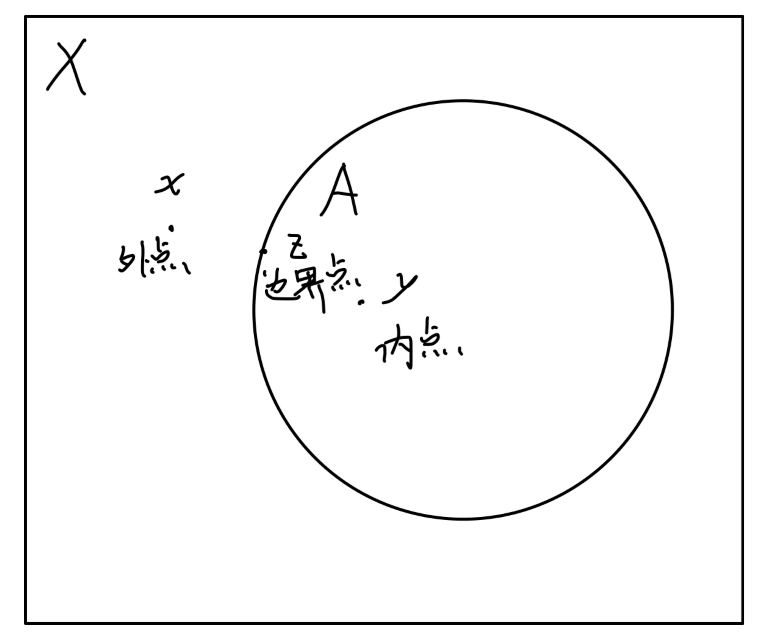## 拓扑

• 包含空集 $\empty$$X$ 自身
• $U$ 中有限个子集的交也属于 $\mathscr{U}$
• $U$ 中任意多个子集的并也属于 $\mathscr{U}$

$\mathscr{U}$$X$ 的一个 拓扑，二元组 $(X,\mathscr{U} )$ 称为 拓扑空间 ，通常可以简写为 $X$$\mathscr{U}$ 的子集称为这个拓扑空间的 开集（open sets）

$\mathscr{U} = \{\empty,X\}$$X$ 的一个拓扑，称为平庸拓扑。

$\mathscr{U} = 2^X$ 显然也是 $X$ 的一个拓扑，称为离散拓扑 (discrete)。

$\mathscr{U}_1$$\mathscr{U}_2$ 是集合 $X$ 上的两个拓扑。若 $\mathscr{U}_1 \subset \mathscr{U}_2$，则称 $\mathscr{U}_1$$\mathscr{U}_2$ 粗。很显然，平庸拓扑最粗，离散拓扑最细。若同时有 $\mathscr{U}_1\subset \mathscr{U}_2,\mathscr{U}_2\subset \mathscr{U}_1$，则称这两个拓扑是 相当的（comparable）

$\mathscr{U} = \{\empty,\{a\}\}$

\begin{aligned} \mathscr{U}_1 &= \{\empty,\{a,b\}\}\\ \mathscr{U}_2 &= \{\empty,\{a,b\},\{a\}\}\\ \mathscr{U}_3 &= \{\empty,\{a,b\},\{b\}\}\\ \mathscr{U}_4 &= \{\empty,\{a,b\},\{a\},\{b\}\}\\ \end{aligned}

### 拓扑的一些重要概念

$\mathscr{U} = \{\empty,\{a,b,c,d,e\},\{a\},\{c,d\},\{a,c,d\},\{b,c,d,e \}\}$

$\{a,b,c,d,e\},\empty,\{b,c,d,e\},\{a,b,e\},\{b,e\},\{a\}$

$x \in X$，点 $x$ 的邻域 $N(x)$$X$ 的一个子集，且包含了一个包含点 $x$ 的开集。类似的也可以定义某个子集的邻域。• $x \in X$ 称为 $A \subset X$内点，若存在一个开集 $U$ 使得 $x \in U \subset A$。子集 $A$ 所有内点形成的集合，记为 $\mathrm{Int} (A)$，并称为 $A$内部 (interior)。它是 $A$ 中最大的开集。
• $x \in X$ 称为 $A \subset X$外点，若其是 $A$ 的补集的内点。即 $x \in \mathrm{Int}(X\setminus A)$。所有外点的集合记为 $\mathrm{Ext}(A)$
• $x \in X$ 称为 $A \subset X$边界点，若它既不是内点也不是外点。$A$ 的所有边界点形成的集合记为 $\dot{A}$

\begin{aligned} \mathrm{Int}(A) &= A'\\ \mathrm{Ext}(A) &= X\setminus A''\\ \dot{A} &= A'' \setminus A'\\ \end{aligned}

$x \in X$ 称为集合 $A \subset X$ 的极限点，如果 $x$ 的每一个邻域 $N(x)$ 至少包含一个不同于它自身的属于 $A$ 的点。即：

$(N(x) - {x}) \cap A\neq \empty ,\forall N(x)$

$A$ 和它的所有极限点的并称为 $A$ 的闭包，记为 $\bar{A}$

$\bar{A}$ 是包含 $A$ 的最小闭集。$A$ 的闭包也是包含 $A$ 的所有闭集的交。

\begin{aligned} \overline{\{b,c,d\}} &= \{b,c,d,e\}\\ \overline{\{a\}} &= \{a\}\\ \overline{\{a,c\}} &= \{a,b,c,d,e\}\\ \end{aligned}

### 可分性

“五元素集合拓扑空间” 不是 Hausdorff 的，因为 $d,c$ 不满足 $T_2$ 可分要求。• $T_0$ 可分
拓扑空间 $X$ 称为 $T_0$ 可分的，若对 $X$ 中的任意两点 $x$$y$，存在一个开集 $U$ 使得 $x \in U$$y \notin U$， 或者 $y \in U$$x \notin U$

• $T_1$ 可分
拓扑空间 $X$ 称为 $T_1$ 可分的，若对 $X$ 中的任意两点 $x$$y$，存在开集$U$$V$ 使得 $x \in U$$y \notin U$，以及 $y \in V$$x \notin V$

• $T_2$ 可分
拓扑空间 $X$ 称为 $T_2$ 可分的，若对 $X$ 中的任意两点 $x$$y$，存在两个不相交的开集 $U$$V$ 使得 $x \in U$，以及 $y \in V$

### 紧致性

$\{U _ i \}$ 中的子集抽出一部分形成一个子系统。若这个子系统也形成 $X$ 的一个覆盖，则称这个覆盖为 $\{U _ i \}$ 的子覆盖。

Hasudorff 空间 $X$ 称为是仿紧的，如果它总存在这样的开覆盖 $\{U_i\}$$X$ 中的任意一点都只被有限个 $U_i$ 覆盖。